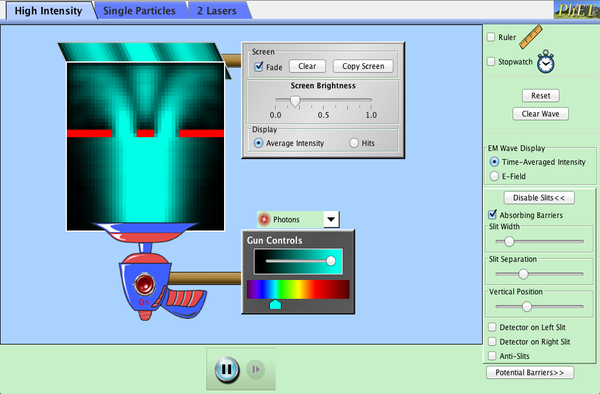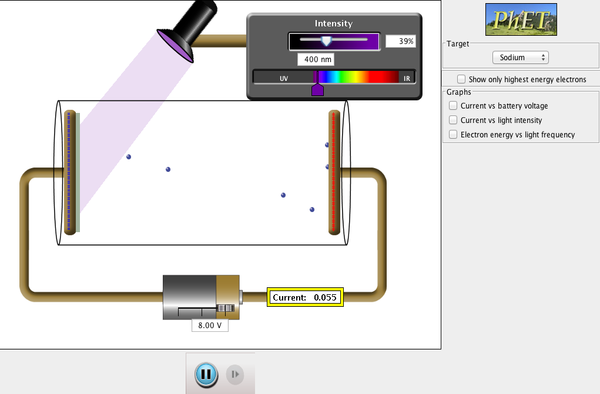## Introduction

The research of part of the theoretical physics group require the use of supercomputers to solve equations. Currently classical computers are used. There is a lot of work on developing "quantum computers", which use quantum mechanics to speed up many calculations. The student will investigate the possibilities of using quantum computers rather than classical computers and report back to the group on what they found out.

The goal of these exercises is for the student to gain enough familiarity with quantum mechanics to use the simulators  of a quantum computer. We will investigate the quantum computer from IBM. The student will also learn the basics of the underlying mathematics of quantum mechanics, such as complex numbers.

There are many exciting possible applications of quantum computing, if they can be built. See this article in Time magazine. Possible applications are:
• decoding some current encryption algorithms, to enable reading of terrorist's communication
• developing better encryption algorithms
• In principle quantum computers will use less energy than classical computers.
• Faster algorithms for machine learning
• Allows simulations of much bigger physical simulations
The video below gives an overview of the basic ideas of quantum computing.

To learn about quantum physics we will use a number of simulations and background material.

### Basic ideas of quantum physics

There are a number of key ideas in quantum physics

• Energy levels can be both continuous and discrete.  In classical physics, the energy of a system is not discrete.
• Particles, such as electrons,  have both particle and wave properties.
• Light has both wave like properties, but it also behave like particles, known as photons.
• The calculations are done in terms of probabilities.

Important back ground topics to quantum mechanics are waves and  complex numbers.

See, the Nobel Prize winner,  Richard Feynman discuss quantum physics. Feynman was one of the first people t think about using quantum mechanics for computation.

## Experimental foundations of quantum mechanics

The theory behind quantum mechanics can be developed from a mathematical viewpoint. However, it is useful to see some of the concepts in quantum mechanics in their original experiments (although you will use computer simulations to investigate the experiments).

### Discrete light spectral lines

This exercise investigates the discrete lines in the spectrum of the lamps. This video shows a real experimental set up, note however that Thomson's idea of an atom as  a plum pudding is no longer correct.

You can download the java based simulation from here.  See the video instructions on using the application. The details of the exercises are here.   The majority of the simulation codes are written in java, so may require some configuration of your computer.

As background information to the application you can read some of the videos from
the Khan academy on quantum physics.

Some specific short lectures (each of length roughly 10 minutes) are:

### Quantum interference

This exercise is based on the wave function application from PHet. This requires java to run.

• As background to particles behaving as a wave you can see this short video.## The Photoelectric effect

• The photoelectric effect was the first evidence that the photon was a particle
• See the video below, from the Khan academy, about the photoelectric effect.### Mathematical background to quantum mechanics

The language of quantum mechanics involves complex numbers and matrices from mathematics. A good review of the formulation is in the book below.  This section provides supplementary background information to the  formalism of quantum physics. There are a variety of different ways of formulating quantum mechanics. The best formalism is Heisenberg matrix mechanics.

Quantum Mechanics: The Theoretical Minimum

by Leonard Susskind and Art Friedman.

### Chapter 1

• The chapter starts with a model of quantum mechanics
• The idea of a Qubit is introduced
• Complex numbers are briefly introduced. There is a more detailed introduction here.
• The chapter ends with a review of vectors.
As background information there is

## Quantum Computing

One important application of quantum mechanics is to the building of quantum computers

### Basic ideas of  classical computers

• A digital computer is based on a string of 1 and 0s at the lowest level
• The basis of a classical computer are logic gates: NOT, AND, OR, NAND. See this discussion from the BBC.

## Basic ideas of quantum computers

In a quantum computer, the 0s and 1s are replaced by Qubits and the logic gates are replaced by quantum gates.

### Background to quantum computing

See the lectures by Michael Nielsen on quantum computing:

Lecture 1: The qubit

Lecture 2, Tips for working with qubits

Lecture 2, The quantum not gate

## IBM quantum experience

Conceptual Quantum Mechanics